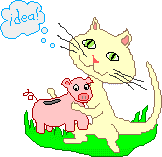IDEA BANKgreat math activities and resources suggested by teachers*1) making the most of online math explorations: - (with links to lots of great math sites)
2) approaches to math instruction
3) math activities for families, math carnivals, math nights, and classroom math centers
4) a math toolbox in every home - (help students make and use math manipulatives)
5) math and literature
7) place value
8) odd and even
9) rounding
10) multiplication
11) order of operations
12) fraction activities  and a collection of sites for exploring fractions
13) geometry and math art
14) resources: geometric construction sets
15) winter math
16) spring math
17) fall math

There will be more Idea Banks coming soon!

* Many of these ideas have been shared by teachers for teachers at "teachers.net" and are used with permission, whenever it was possible to contact the authors.Search WWW
Search Math Cats for Teachers and Parents with Google!